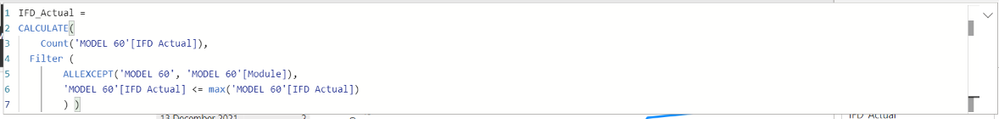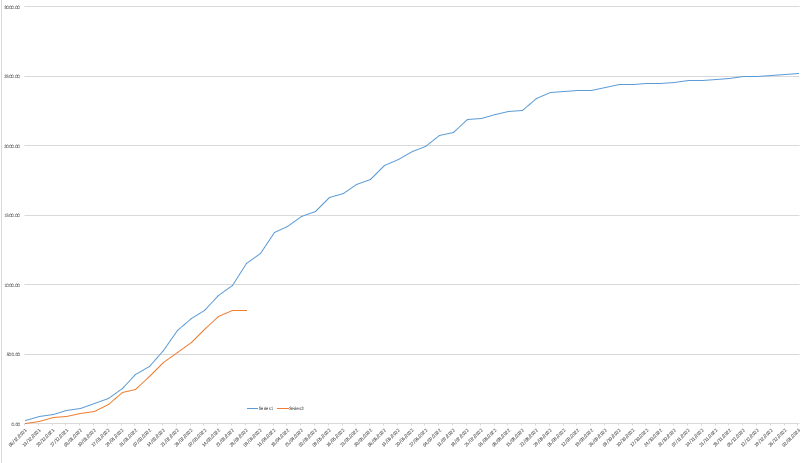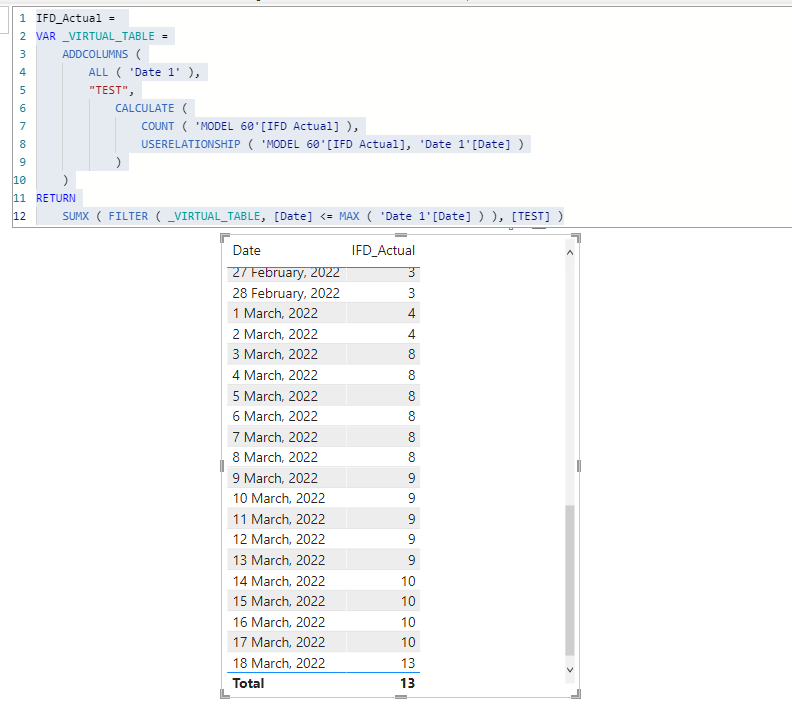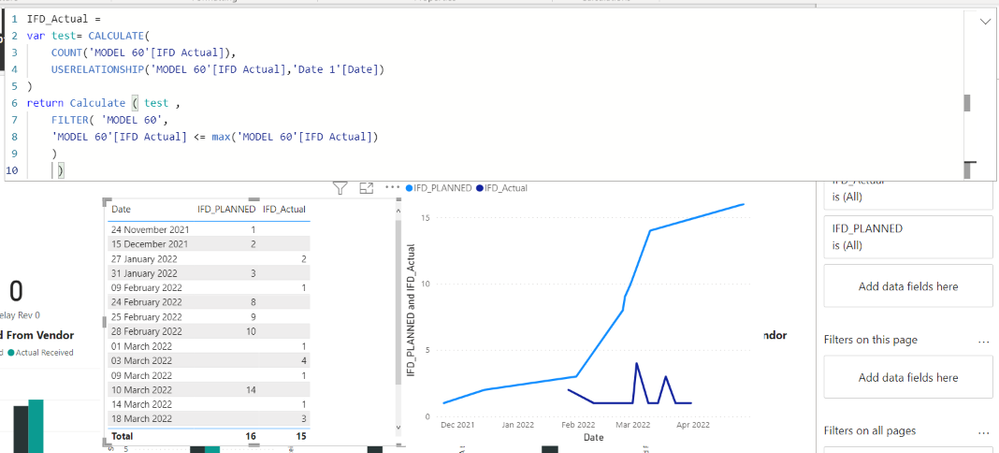cancel
Showing results for
Did you mean:Anonymous
Not applicable

## Merge in a single Line Chart two visuals with different dates columnsDear PowerBi Community,

I would like to share you a problem that I'm facing right now:

I have two differents columns dates (IFD Actual and IFD Planned) that after counting them  (Dax formule in the 3rd picture), I would like to show in the same graph as I was capable to do in Excel. I tried to use one unique calendar table with USERELATIONSHIP without any particular success. Last remark: the two graphs separetely show the correct information as you may see in the second picture.

1 ACCEPTED SOLUTIONCommunity Support

Hi @Anonymous ,

I suggest you to create a virtual table in var and then calculate the running total.

My measure:

``````IFD_Actual =
VAR _VIRTUAL_TABLE =
ALL ( 'Date 1' ),
"TEST",
CALCULATE (
COUNT ( 'MODEL 60'[IFD Actual] ),
USERELATIONSHIP ( 'MODEL 60'[IFD Actual], 'Date 1'[Date] )
)
)
RETURN
SUMX ( FILTER ( _VIRTUAL_TABLE, [Date] <= MAX ( 'Date 1'[Date] ) ), [TEST] )``````

In my sample, [Date] column in my table visual is from 'Date 1'. So the filter in sumx is [Date] (from virtual table) <= MAX('Date1'[Date]).

Note: We need to add the [Date] column in table visual in Max field in sumx filter.Best Regards,
Rico Zhou

If this post helps, then please consider Accept it as the solution to help the other members find it more quickly.

4 REPLIES 4Super User

@Anonymous , with inactive join you need to create measure

calculate(countrows(table),
USERELATIONSHIP ( 'Table'[IFD Actual], DimDate[Date] )
)

calculate(countrows(table),
USERELATIONSHIP ( 'Table'[IFD Planned], DimDate[Date] )
)

https://community.powerbi.com/t5/Community-Blog/HR-Analytics-Active-Employee-Hire-and-Termination-trend/ba-p/882970Anonymous
Not applicable

Dear @amitchandak , thanks a lot for your reply, I tried as you indicated but it doens't work properly:EDIT: I analysed carefully the problem and in the Userelationship I have to use IFD Actual and not IFD Planned as I did, the problem is that with IFD Actual , due to the filter that I'm using, the final graph is empty. So I have to find another way to do the same thing that the filter doesAnonymous
Not applicableI'm close, I need only that the IFD actual count has a cumulative functionCommunity Support

Hi @Anonymous ,

I suggest you to create a virtual table in var and then calculate the running total.

My measure:

``````IFD_Actual =
VAR _VIRTUAL_TABLE =
ALL ( 'Date 1' ),
"TEST",
CALCULATE (
COUNT ( 'MODEL 60'[IFD Actual] ),
USERELATIONSHIP ( 'MODEL 60'[IFD Actual], 'Date 1'[Date] )
)
)
RETURN
SUMX ( FILTER ( _VIRTUAL_TABLE, [Date] <= MAX ( 'Date 1'[Date] ) ), [TEST] )``````

In my sample, [Date] column in my table visual is from 'Date 1'. So the filter in sumx is [Date] (from virtual table) <= MAX('Date1'[Date]).

Note: We need to add the [Date] column in table visual in Max field in sumx filter.Best Regards,
Rico Zhou

If this post helps, then please consider Accept it as the solution to help the other members find it more quickly.